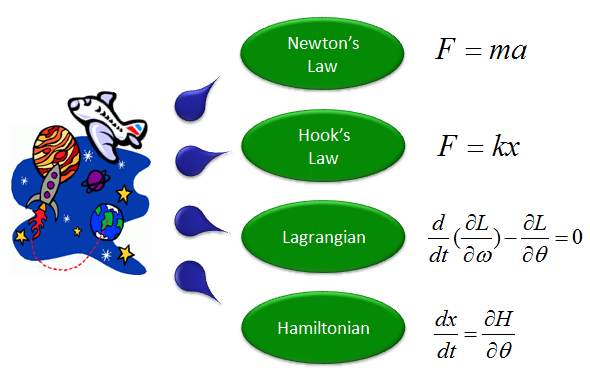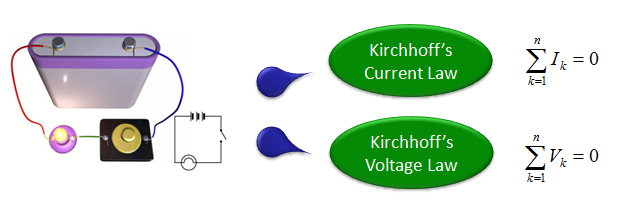DE - Modeling                                                                          Home : www.sharetechnote.com How to get Governing Equation   You will notice from the sample models in later sections that the most important step for modeling is to figure out 'Governing Equation' for the system. Then you may ask "How can I get the governing equation for the system ?". The simplest answer would be "use your common sense or intuition and try to make your own question about the system". For example, let's suppose that you have a mass tied to the end of a spring in vertical direction and pulled the mass down and released it. Possible questions for this case would be Where the mass will be at 2 seconds from now ? What would be the speed of the mass at 2 seconds from now ? This is a good starting point, but these question by themselves does not generate the governing equations. You need some formal knowledge to figure out the governing equation that will give you the answers to your questions.   There are several useful rules that can lead you to a geverning equation. In case of mechanical system/dynamic system (system dealing with moving object), followings can be the most frequently used rules. Don't try just blindly memorize these rules and it would not help much. Just try to understand the meaning of these rules. I would not explain any further about these equation. Try yourself to find materials from internet or text book. Try reading different sources as diverse as possible. Everybody would give you a little bit different aspect and insight. As you read through those sources, your brain would reorgnaize it by itself and you will get your own understanding of these rules. If you have solid understanding of these rules, you would be able to follow through the example models in this post without any difficulties.If you are dealing with any electrical/ectronic system, following rules can be a good source that lead you to a proper governing equation.